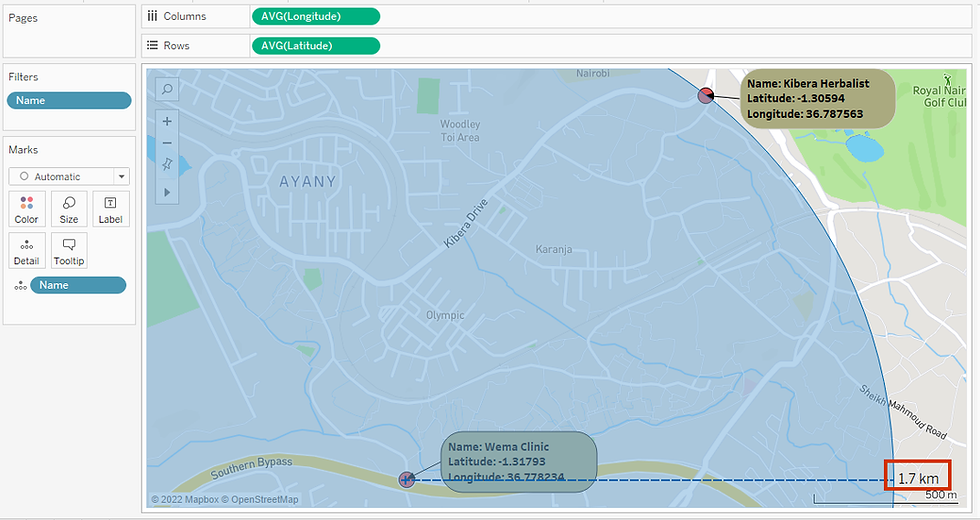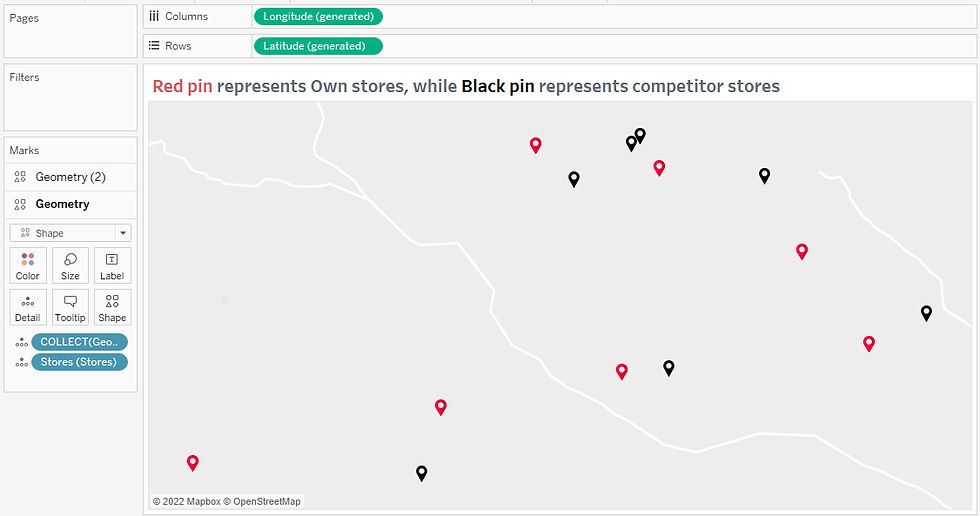top of page
Search
• Bernard K

# Tableau Spatial FunctionsSpatial functions are used to perform advanced spatial analysis in Tableau. In this article, I am going to demonstrate various ways you can apply spatial function in real-life data visualization examples.

Particularly in this article, you’ll learn how to use the following spatial functions.

• AREA () – a function used to compute the total surface area of a spatial polygon.

• BUFFER () – a function used to create a boundary around a spatial point - as a way of measuring distance between two points.

• DISTANCE () - a function used to measure distance between two points in a specified unit.

• MAKELINE () – a function used to generate line mark between two points. Useful when building origin-destination maps.

• MAKEPOINT () - a function used to convert latitude and longitude columns into spatial objects.

### Application of AREA () function in Tableau

The syntax

AREA (polygon, units)

AREA ([Geometry], ‘km’) – which computes the total surface area of a spatial polygon in kilometers.

AREA ([Geometry],’mi’) – which computes the total surface area of a spatial polygon in miles.

For example, the map below shows the subnational administrative boundaries for Egypt, created using polygons.(For the purpose of your analysis, you may be interested in the computing the surface area of each of these administrative boundaries – something which you can achieve using the AREA function as shown below)Adding the two calculations to the view returns the total surface area of each administrative boundary in the specified units.### Application of MAKEPOINT () function in Tableau

The syntax.

MAKEPOINT (number, number) or MAKEPOINT (number, number, number)

e.g., MAKEPOINT ([Latitude], [Longitude]) – which returns a spatial object constructed from the latitude and longitude fields.

For example, let’s say you’ve sample data like the one shown in the snapshot below.You can plot the various facilities in this data by first creating a spatial object using the latitude and longitude fields as shown below.Using the above calculation ‘Spatial Object’, you can plot all the facilities in the data by dragging the field to the detail shelf.(Although you can plot the same data points by dragging the Latitude and Longitude fields to the rows and columns shelf. The above technique comes in handy when plotting multiple map layers in Tableau)

### Application of DISTANCE () function in Tableau

The syntax.

DISTANCE (Start, End, Units)

e.g., DISTANCE (MAKEPOINT (Latitude, Longitude), MAKEPOINT (Latitude, Longitude), ‘km’)

For example, one can compute the distance between the two facilities in this map using the formula below.DISTANCE (MAKEPOINT (-1.31793,36.778234), MAKEPOINT (-1.30594,36.787563), ’km’) – which computes the distance between the two facilities in Kilometers.

(The above formula returns the distance between the two facilities as 1.6839Km = approximately 1.7km)

This is the same result you’ll get when you measure the same distance using the radial selection option under the map view toolbar.### Application of MAKELINE () function in Tableau

The syntax.

MAKELINE (Start, End)

E.g., MAKELINE (MAKEPOINT (Latitude, Longitude), MAKEPOINT (Latitude, Longitude)) – which will draw a line between the two data points.

For example, you may want to draw a line connecting the two facilities in the map below.You can achieve that using the formula below.Dragging the above calculation to the Marks Layer.Will automatically draw a line connecting the two facilities. A technique most useful when building origin-destination maps.### Application of BUFFER () function in Tableau

The syntax.

BUFFER (geometry, number, units)

e.g., BUFFER (MAKEPOINT (Latitude, Longitude), 4, ‘km’) – which returns a 4km boundary around a point.

For example, a business may want to find out how many competitor stores are within 250 ft of their stores.

Using the sample data in the snapshot below.One can answer the above question as follows.

Using multiple map layer feature, create a map plotting own stores against competitor stores as shown below.Next create a buffer calculation using geolocation data to return a 250 ft boundary around the stores, using the calculation below.Drag the above calculation to the Marks Layer to plot the boundaries.Executing this we’ve.(From this view, you can see which of the competitor stores are within 250 ft of own stores)